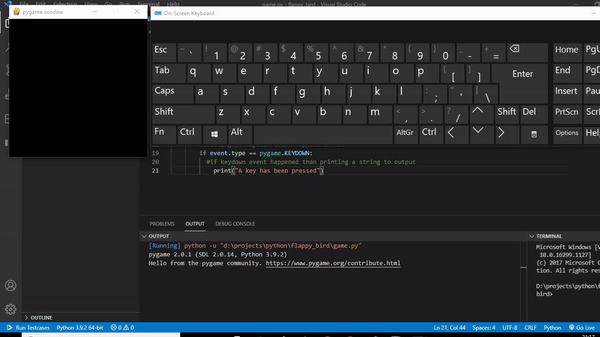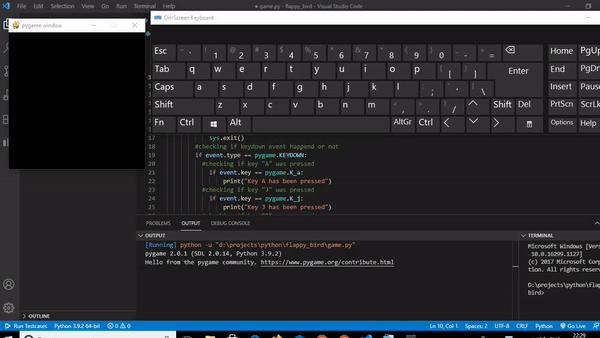# How to get keyboard input in PyGame ?

• Last Updated : 08 Jun, 2022

While using pygame module of Python, we sometimes need to use the keyboard input for various operations such as moving a character in a certain direction. To achieve this, we have to see all the events happening. Pygame keeps track of events that occur, which we can see with the events.get() function. In this article, we are going to discuss how we can get and use various keyboard inputs in pygame.

### Detecting if a key is pressed:

Whenever a key is pressed or released, pygame.event() queue methods pygame.KEYDOWN and pygame.KEYUP events respectively.

For example, if we want to detect if a key was pressed, we will track if any event of pygame.KEYDOWN occurred or not and, accordingly, we will get to know if any key was pressed or not. The code for detecting if any key was pressed or not can be written as:

## Python

 `# importing pygame module``import` `pygame` `# importing sys module``import` `sys` `# initialising pygame``pygame.init()` `# creating display``display ``=` `pygame.display.set_mode((``300``, ``300``))` `# creating a running loop``while` `True``:``      ` `    ``# creating a loop to check events that``    ``# are occurring``    ``for` `event ``in` `pygame.event.get():``        ``if` `event.``type` `=``=` `pygame.QUIT:``            ``pygame.quit()``            ``sys.exit()``        ` `        ``# checking if keydown event happened or not``        ``if` `event.``type` `=``=` `pygame.KEYDOWN:``          ` `            ``# if keydown event happened``            ``# than printing a string to output``            ``print``(``"A key has been pressed"``)`

Output:After running this code, it is seen that whenever a key has pressed a string “A key has been pressed” is printed on the terminal

### Detecting which key was pressed:

To know which key was pressed, we have to check the event.key variable corresponds to which pygame keys. For example, the pygame key for the letter “A” is “K_a” then we will compare event.Key with K a and if it comes to be same that means the key “A” was pressed.

The various keyboard key and corresponding pygame keys are:

For example, let’s create a code to check if key “A” or “J” or “P” or “M” was pressed or not. The code for checking will be:

## Python

 `# importing pygame module``import` `pygame` `# importing sys module``import` `sys` `# initialising pygame``pygame.init()` `# creating display``display ``=` `pygame.display.set_mode((``300``, ``300``))` `# creating a running loop``while` `True``:``      ` `    ``# creating a loop to check events that``    ``# are occurring``    ``for` `event ``in` `pygame.event.get():``        ``if` `event.``type` `=``=` `pygame.QUIT:``            ``pygame.quit()``            ``sys.exit()``        ` `        ``# checking if keydown event happened or not``        ``if` `event.``type` `=``=` `pygame.KEYDOWN:``              ` `            ``# checking if key "A" was pressed``            ``if` `event.key ``=``=` `pygame.K_a:``                ``print``(``"Key A has been pressed"``)``              ` `            ``# checking if key "J" was pressed``            ``if` `event.key ``=``=` `pygame.K_j:``                ``print``(``"Key J has been pressed"``)``              ` `            ``# checking if key "P" was pressed``            ``if` `event.key ``=``=` `pygame.K_p:``                ``print``(``"Key P has been pressed"``)``            ` `            ``# checking if key "M" was pressed``            ``if` `event.key ``=``=` `pygame.K_m:``                ``print``(``"Key M has been pressed"``)`

Output:When we run this code and press the given keys the corresponding strings will be printed on the terminal.

My Personal Notes arrow_drop_up